# Machine Learning Glossary: A-2-Z Terms In Machine Learning

Accuracy:- Accuracy is used to evaluate a classification model. It is defined as the percentage of the total number of correct predictions. Mathematically it is represented as:Algorithm:- In Machine Learning, Algorithm is the procedure applied to the data to create a machine learning model. e.g., Linear Regression, Decision Trees.

Annotation:- Process of labeling the data or in more specific terms it is the task of labeling the data using metadata for training a machine learning model

Artificial Neural Networks:- Machine Learning Algorithms inspired from biological neural networks that constitute animal brain cells.

Attribute:- An aspect of an instance. In terms of Excel Sheet, the column's name is Attribute, e.g., Colour, Temperature, etc.

AUC (Area Under Curve):- Area Under ROC Curve represents the classification model's aggregate performance for all classification thresholds.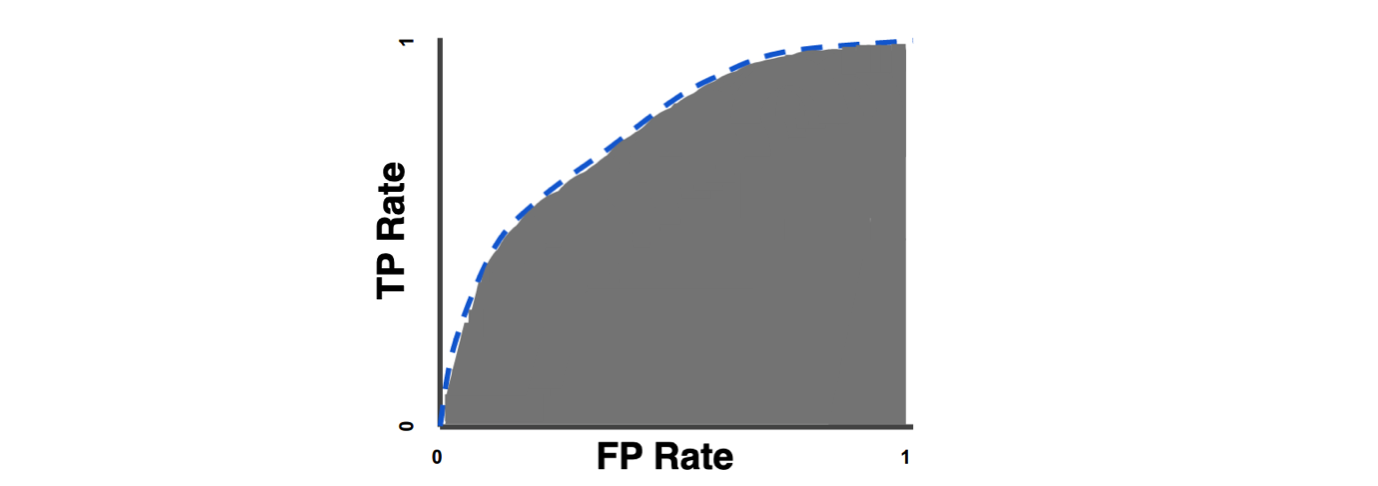Bias:- Bias is known as the difference between the predicted value and the true value. It allows the machine learning model to learn the offset present while mapping the input and output of the model. For example, if all input features are at origin, will the result also be at the origin? Can there be some base value upon which features affect?

Bias Error:- Error caused by algorithm’s tendency to consistently learn the wrong thing by not taking into account all the data's information.

1. High Bias:- Tendency of making assumptions about the data becomes higher, and hence error increases.
2. Low Bias:- Tendency of making assumptions about the data becomes lesser. Model Learns accurately on training data.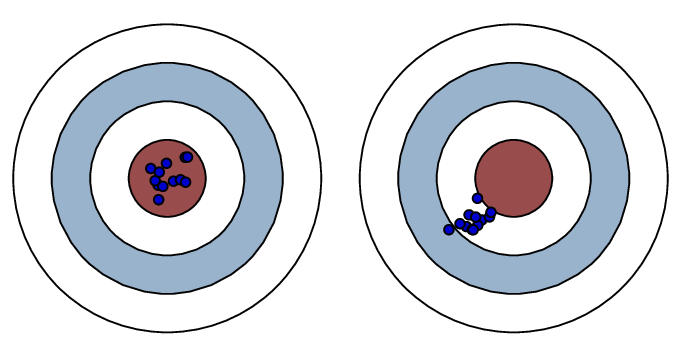Image: Low Bias High Bias

Classification:- A type of problem in Machine learning where machine learning models try to predict the category of output.

1. Binary Classification:- Classifies input in two binary classes; for example, an image contains a cat in it or not.
2. Multi-label Classification:- Classifies objects according to the multiple labels. For example, Image detects the presence of House, Cat, Dog, etc., simultaneously.

Classification Threshold:- It is the limiting value based on which a particular decision is made. Suppose if a machine learning model predicts Cat's presence in any image with the surety of X%. Suppose you have set criteria that if confidence > 60%, then that will be a valid prediction. Then the threshold value is 60 for the classification.

Clustering:- A type of unsupervised learning where the model groups the input data into different buckets based on some inherent features of the data. Generally, clusters consist of the item having similar characteristics. Some of the most commonly used clustering algorithms are KMeans, Hierarchical, Affinity Clustering.

Confusion Matrix:- A metric for performance measurement of machine learning classification problem where output can be two or more classes. It groups the prediction into four categories,

1. True Positive:- Image of Cat classified as Image of Cat by the machine learning model
2. True Negative:- There was no cat in the Input Image, and the Machine learning model also predicted no cat.
3. False Positive:- Image of Dog is classified as Image of Cat by ml model. This is also called Type I Error.
4. False Negative:- There was a cat in Input Image, but the Machine learning model predicted no cat. They are also called Type II Error.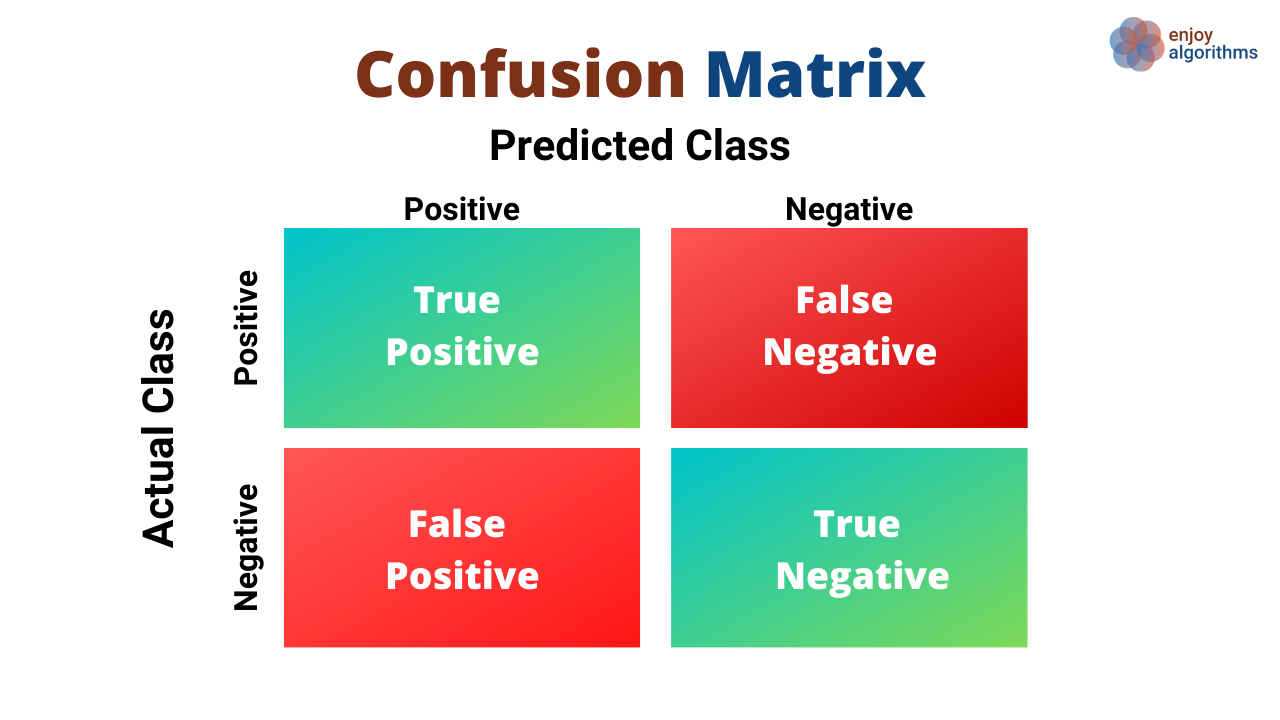Convergence:- A state during training a machine learning model when a change in the loss values becomes less between consecutive epochs or, more specifically, if the change in the cost of the loss function is very minute, then it could be said that the model has found the minima or its position will not change further, i.e., it has converged.

Deep Learning:- A subfield of machine learning which deals with algorithms based on Artificial Neural Networks. It is also known as deep structured learning.

Dimension:- Dimension in machine learning means the number of features that have been used as Inputs for the machine learning Algorithms.

Dropout:- A type of regulariser used to prevent over-fitting by dropping out hidden or visible unfits while training neural networks.

Epoch:- 1 Epoch = 1 iteration over entire dataset.

Extrapolate:- A type of estimation beyond the original observation range.

False Positive Rate (FPR):- FPR is the probability of falsely rejecting the null hypothesis for a particular test. Mathematically it is calculated as:Feature:- Combination of attribute type and its value is known as a feature. Temperature is Attribute, and Temperature = 25 Degrees is a feature.

Feature Vector:- A list of all the features included as an Input to the ML model. In terms of Excel, A row is Feature Vector.

Global Minima:- The Value of loss function reached a minimum globally over the entire loss function domain. It is the smallest overall value of a function over its entire range.

Hidden Layers:- Layers in-between the Input and Output Layers in a neural network are hidden layers.

Hyperparameters:- A parameter whose value is used to control the learning process. E.g., The number of hidden layers in a Neural Network.

Instance:- A sample, row of feature value in the dataset. It is also called Observation.

i.i.d. sample:- It means each random variable of the sample has the same probability distribution, and all are mutually independent.

Label:- The output data that is used in the training of a supervised learning model. e.g., To train a Cat Classifier Model, we need to prepare a dataset in which we label the image by saying that it is cat or not-cat.

Learning Rate:- A tuning parameter in any optimization problem which determines the step-size at each epoch while moving towards any minima (Global / Local ) of the loss function.

Loss:- In simple terms, Loss = (Actualvalue) - (Predictedvalue)
Lower the loss value, better the model (Unless overfitted)

Local Minima:- The value of the loss function becomes minimum at that point in a local region. It is a point where the function value is smaller than at nearby points but possibly greater than at a distant point.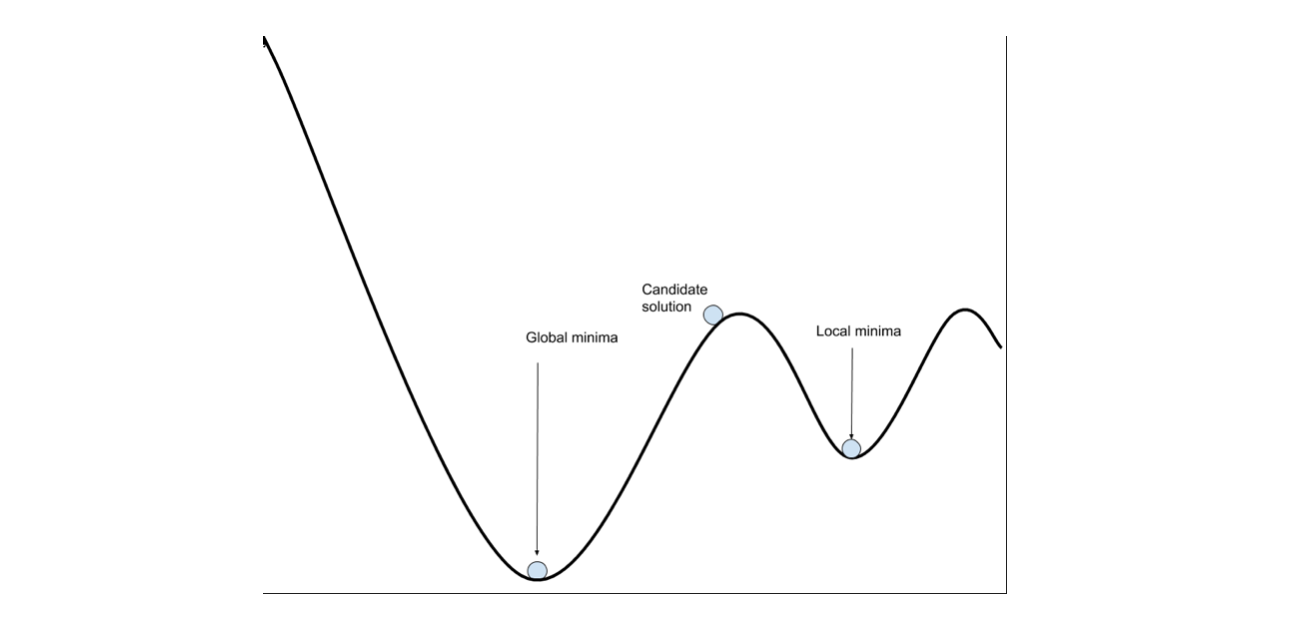Machine Learning:- A computer science field that gives computers the ability to learn without being explicitly programmed.

Model:- Model is the output of any ML algorithm that is run on the data.

Neural Networks:- Machine Learning algorithm inspired from biological neural networks which constitute animal brain cells.

Normalization:- Rescaling feature values to constrain dataset values to a standard range in any regression problem. It improves computation speed.

Noise:- Additional meaningless information present in the data.

Null Accuracy:- Accuracy can be achieved by directly predicting the most frequent class in any classification problem.

Observation:- A sample, row of feature value in the dataset. It is also called an instance.

Optimizers:- Methods that change the value of parameters so that losses reach the minimum. They are used to solve optimization problems by minimizing the function.

Outlier:- Data Samples that differ significantly from other observations.Overfitting:- Situation when model training error becomes significantly less as compared to the model testing error. In this case, the model performs very well on training data but poorly on test data.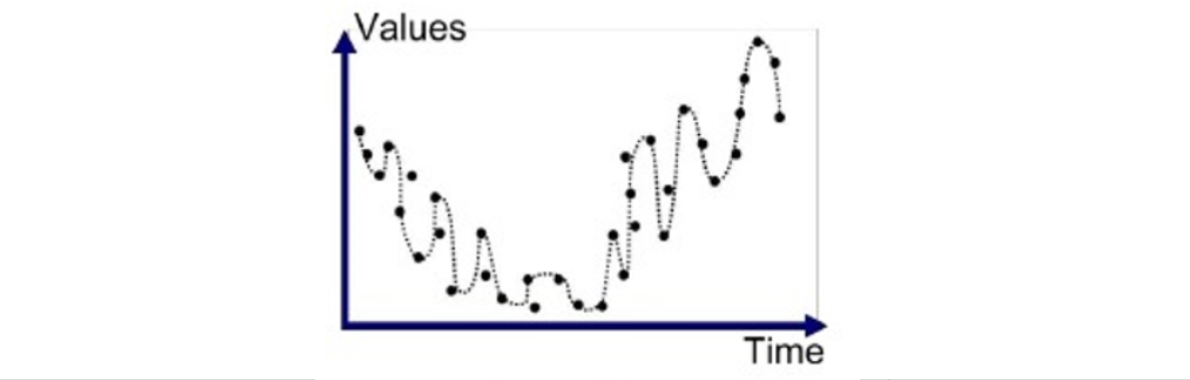Parameters:- Variables whose value we learn from training any machine learning model. e.g., Weights of neural networks.

Precision:- Precision tries to answer the question of,
What portion of True Positive is actually correct?Recall:- Recall tries to answer the question of,
What portion of True Positive is identified correctly?Regression:- A type of machine learning in which prediction output is continuous.

Regularization:- Technique that is used to combat the problem of overfitting.

Reinforcement Learning:- A subset of machine learning in which learning is based upon maximizing the reward based on actions of the model.

ROC (Receiver Operating Characteristic) Curve:- A graph of True Positive Rate vs. False Positive Rate, which is used to check a classification model's performance at different classification thresholds.Sensitivity:-Specificity:-Supervised Learning:- Training a machine learning model under the supervision of a labeled dataset.

Test Set:- Data samples are used to check the generalisability of the machine learning model. These sets are unseen to the model.

Train Set:- Data samples used in training the machine learning model.

Transfer Learning:- Method in which algorithm uses the already trained model to solve the related problem.

True Positive Rate:- Same as Recall.Type 1 Error:- Same as False Positive

Type 2 Error:- Same as False Negative

Underfitting:- A situation in which the machine learning model does not learn the variation present in the data.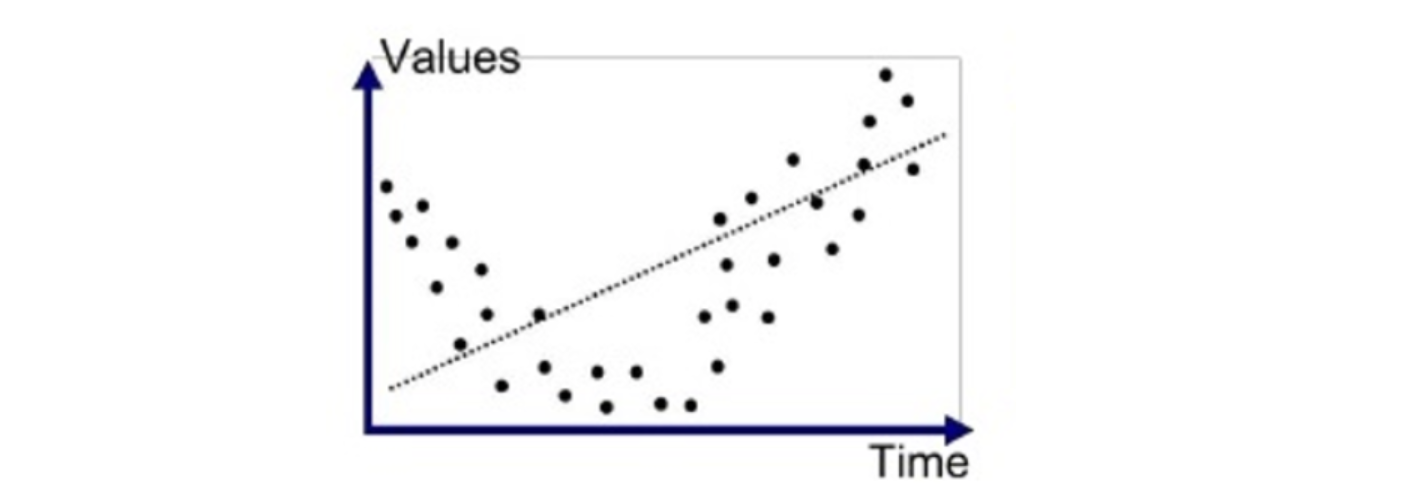Universal Approximation Theorem:- For ANN, if a Model is trained for the input range of (a,b), then the model would be expected to perform well on the test data set, which lies with the range of (a,b) only.

Unsupervised Learning:- A class of machine learning in which training is based upon an unlabelled dataset. E.g., KNN, Clustering.

Validation Set:- Observation used to validate the trained model while training by checking the generalisability of parameters tuned.

Variance:- An error from sensitivity to small fluctuations in the training dataset.

1. Low Variance:- Refers to the situation in which model output varies very little.
2. High Variance:- Refers to the situation in which the model starts following the noise patterns very accurately, and eventually, it overfits the data.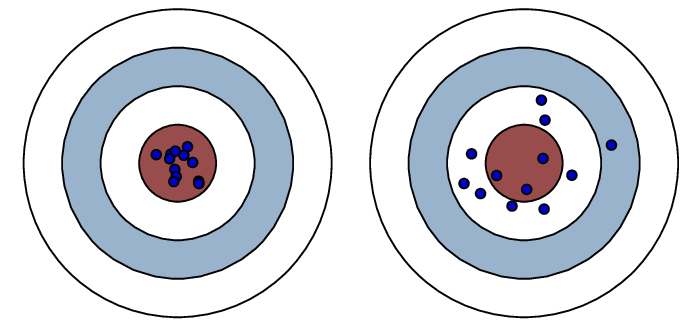Image: low Variance High Variance

Weights:- A learnable parameter in machine learning.

Note: This glossary will keep evolving in the upcoming future.

### Enjoy Learning! Enjoy Machine Learning! Enjoy Algorithms!

Get well-designed application and interview centirc content on ds-algorithms, machine learning, system design and oops. Content will be delivered weekly.